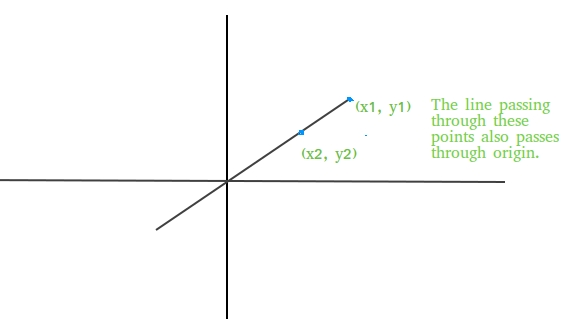# Check if a line passes through the origin

• Difficulty Level : Easy
• Last Updated : 07 Apr, 2021

Given two coordinates of a line as (x1, y1) and (x2, y2), find if the line passing through these points also passes through origin or not.Attention reader! All those who say programming isn't for kids, just haven't met the right mentors yet. Join the  Demo Class for First Step to Coding Coursespecifically designed for students of class 8 to 12.

The students will get to learn more about the world of programming in these free classes which will definitely help them in making a wise career choice in the future.

Examples:

```Input : (x1, y1) = (10, 0)
(x2, y2) = (20, 0)
Output : Yes
The line passing through these points
clearly passes through the origin as
the line is x axis.

Input : (x1, y1) = (1, 28)
(x2, y2) = (2, 56)
Output : Yes```

Approach: Equation of a line passing through two points (x1, y1) and (x2, y2) is given by
y-y1 = ((y2-y1) / (x2-x1))(x-x1) + c
If line is also passing through origin, then c=0, so equation of line becomes
y-y1 = ((y2-y1) / (x2-x1))(x-x1)
Keeping x=0, y=0 in the above equation we get,
x1(y2-y1) = y1(x2-x1)
So above equation must be satisfied if any line passing through two coordinates (x1, y1) and (x2, y2) also passes through origin (0, 0).

## C++

 `/* C++ program to find if line passing through``   ``two coordinates also passes through origin``   ``or not */``#include ``using` `namespace` `std;` `bool` `checkOrigin(``int` `x1, ``int` `y1, ``int` `x2, ``int` `y2)``{``   ``return` `(x1 * (y2 - y1) == y1 * (x2 - x1));``}` `// Driver code``int` `main()``{``    ``if` `(checkOrigin(1, 28, 2, 56) == ``true``)``      ``cout << ``"Yes"``;``    ``else``      ``cout << ``"No"``;``    ``return` `0;``}`

## Java

 `// Java program to find if line passing through``// two coordinates also passes through origin``// or not``import` `java.io.*;` `class` `GFG {``    ` `    ``static` `boolean` `checkOrigin(``int` `x1, ``int` `y1,``                                       ``int` `x2, ``int` `y2)``    ``{``        ``return` `(x1 * (y2 - y1) == y1 * (x2 - x1));``    ``}` `    ``// Driver code``    ``public` `static` `void` `main (String[] args)``    ``{``        ``if` `(checkOrigin(``1``, ``28``, ``2``, ``56``) == ``true``)``            ``System.out.println(``"Yes"``);``        ``else``            ``System.out.println(``"No"``);``    ``}``}` `// This code is contributed by Ajit.`

## Python3

 `# Python program to find if line``# passing through two coordinates``# also passes through origin or not` `def` `checkOrigin(x1, y1, x2, y2):``    ``return` `(x1 ``*` `(y2 ``-` `y1) ``=``=` `y1 ``*` `(x2 ``-` `x1))``    ` `# Driver code``if` `(checkOrigin(``1``, ``28``, ``2``, ``56``) ``=``=` `True``):``    ``print``(``"Yes"``)``else``:``    ``print``(``"No"``)``    ` `# This code is contributed``# by Anant Agarwal.`

## C#

 `// C# program to find if line passing through``// two coordinates also passes through origin``// or not``using` `System;` `class` `GFG {` `    ``static` `bool` `checkOrigin(``int` `x1, ``int` `y1,``                            ``int` `x2, ``int` `y2)``    ``{``        ``return` `(x1 * (y2 - y1) == y1 * (x2 - x1));``    ``}` `    ``// Driver code``    ``public` `static` `void` `Main()``    ``{``        ``if` `(checkOrigin(1, 28, 2, 56) == ``true``)``            ``Console.WriteLine(``"Yes"``);``        ``else``            ``Console.WriteLine(``"No"``);``    ``}``}` `// This code is contributed by vt_m.`

## PHP

 ``

## Javascript

 ``
Output:
`Yes`

My Personal Notes arrow_drop_up